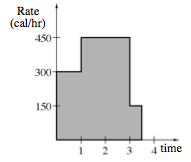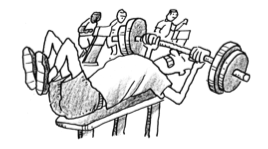### Home > CALC > Chapter Ch2 > Lesson 2.1.2 > Problem2-21

2-21.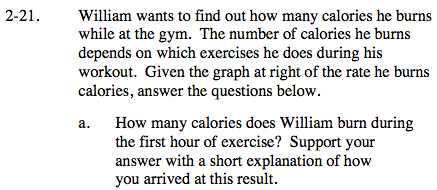Since area of rectangles = (base)(height)on this graph: area = (hours)(calories/hour) = calories. So compute the area under the curve between t = 0 and t = 1.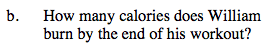Refer to the hint for part (a).

In order to use known geometric formulas, you will have to dissect this problem into parts.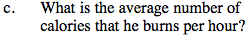$\text{Average calories }= \frac{\text{total calories}}{\text{total time}}$